# 4 APPLICATIONS OF DIFFERENTIATION APPLICATIONS OF DIFFERENTIATION We

• Slides: 84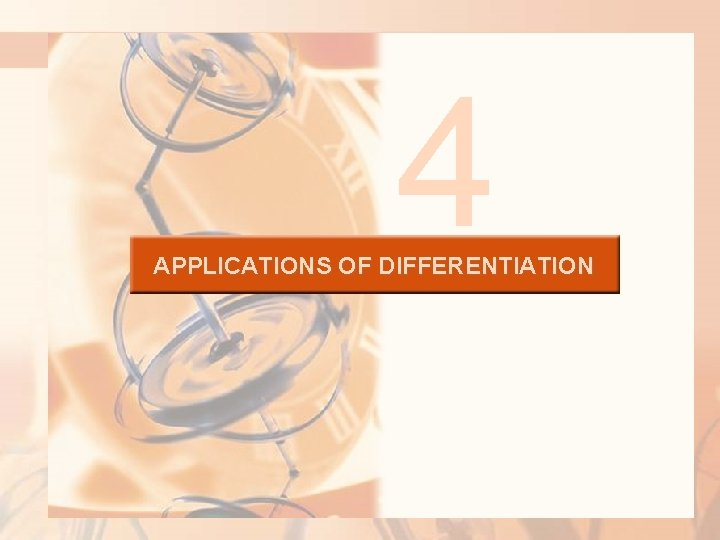4 APPLICATIONS OF DIFFERENTIATION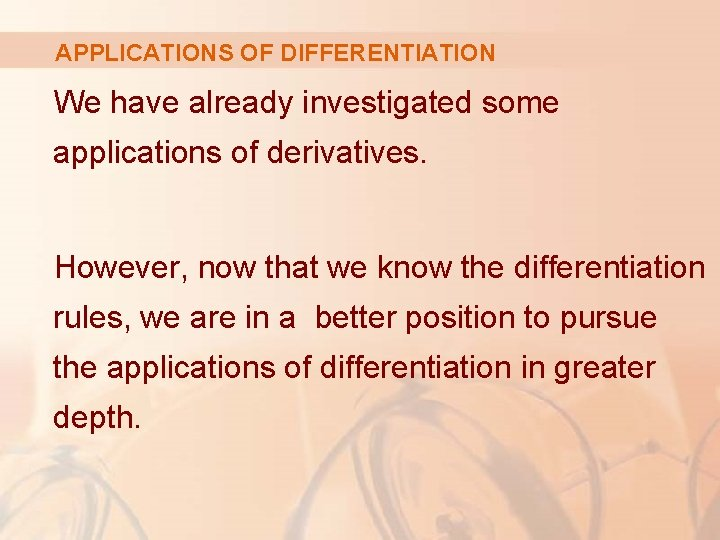APPLICATIONS OF DIFFERENTIATION We have already investigated some applications of derivatives. However, now that we know the differentiation rules, we are in a better position to pursue the applications of differentiation in greater depth.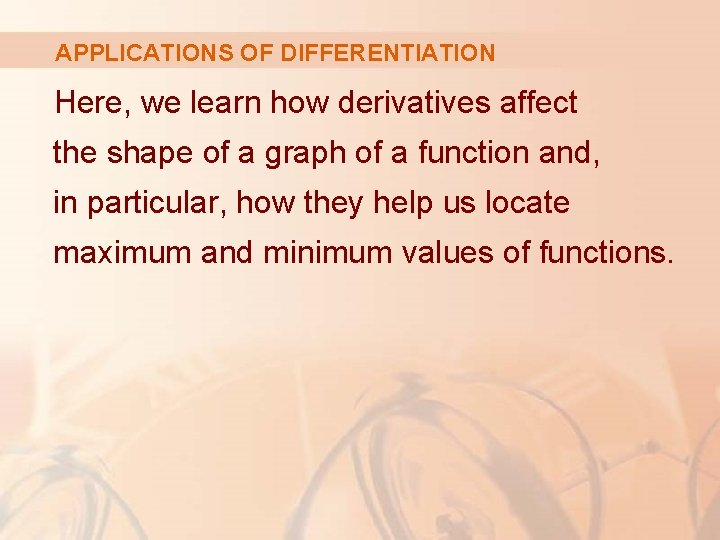APPLICATIONS OF DIFFERENTIATION Here, we learn how derivatives affect the shape of a graph of a function and, in particular, how they help us locate maximum and minimum values of functions.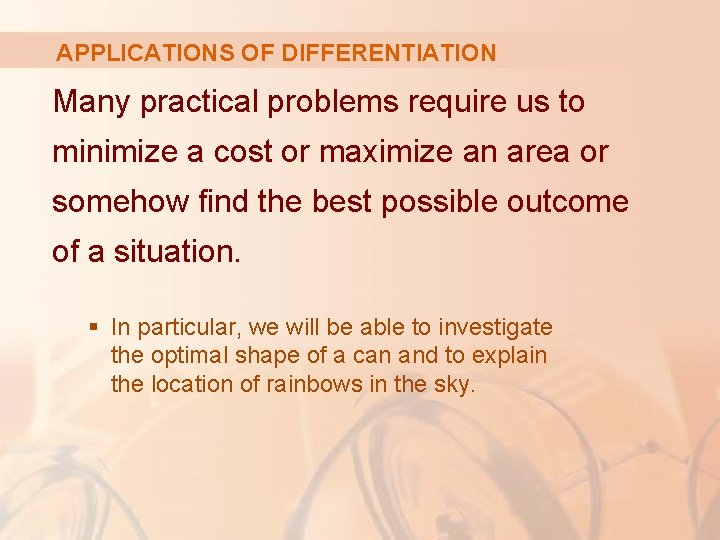APPLICATIONS OF DIFFERENTIATION Many practical problems require us to minimize a cost or maximize an area or somehow find the best possible outcome of a situation. § In particular, we will be able to investigate the optimal shape of a can and to explain the location of rainbows in the sky.APPLICATIONS OF DIFFERENTIATION 4. 1 Maximum and Minimum Values In this section, we will learn: How to find the maximum and minimum values of a function.OPTIMIZATION PROBLEMS Some of the most important applications of differential calculus are optimization problems. § In these, we are required to find the optimal (best) way of doing something.EXAMPLES Here are some examples of such problems that we will solve in this chapter. § What is the shape of a can that minimizes manufacturing costs? § What is the maximum acceleration of a space shuttle? (This is an important question to the astronauts who have to withstand the effects of acceleration. )EXAMPLES Here are some more examples. § What is the radius of a contracted windpipe that expels air most rapidly during a cough? § At what angle should blood vessels branch so as to minimize the energy expended by the heart in pumping blood?OPTIMIZATION PROBLEMS These problems can be reduced to finding the maximum or minimum values of a function. § Let’s first explain exactly what we mean by maximum and minimum values.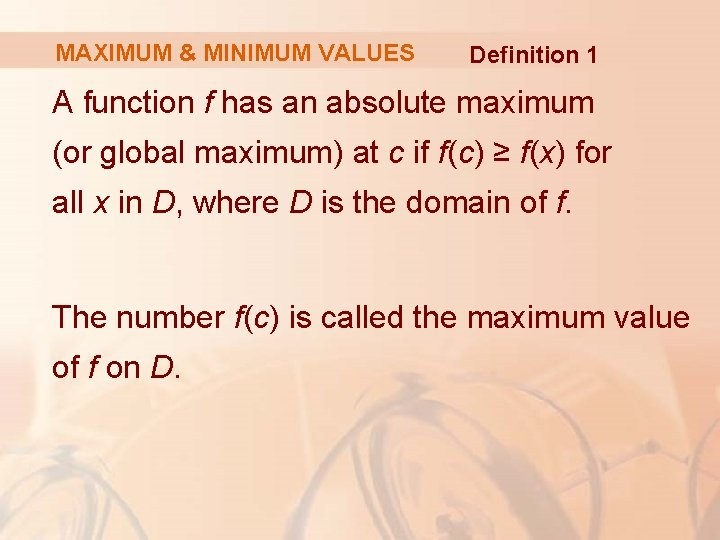MAXIMUM & MINIMUM VALUES Definition 1 A function f has an absolute maximum (or global maximum) at c if f(c) ≥ f(x) for all x in D, where D is the domain of f. The number f(c) is called the maximum value of f on D.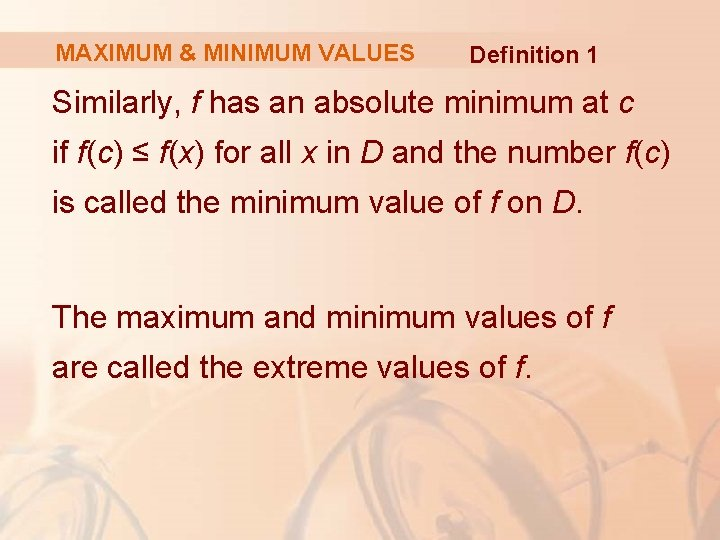MAXIMUM & MINIMUM VALUES Definition 1 Similarly, f has an absolute minimum at c if f(c) ≤ f(x) for all x in D and the number f(c) is called the minimum value of f on D. The maximum and minimum values of f are called the extreme values of f.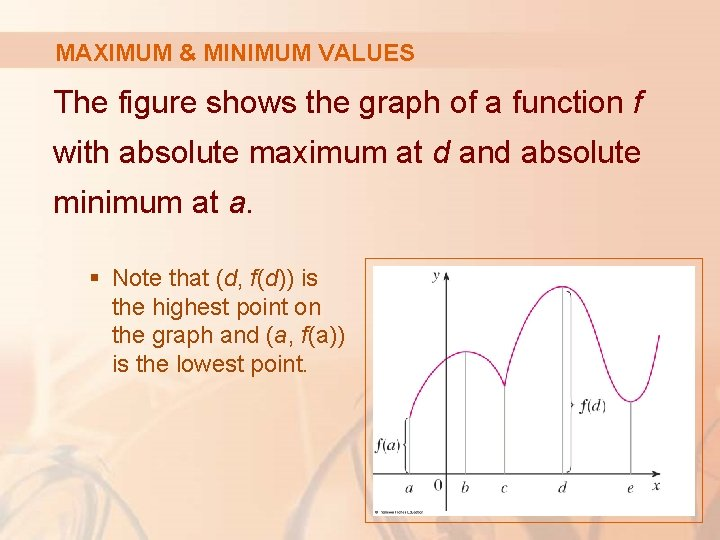MAXIMUM & MINIMUM VALUES The figure shows the graph of a function f with absolute maximum at d and absolute minimum at a. § Note that (d, f(d)) is the highest point on the graph and (a, f(a)) is the lowest point.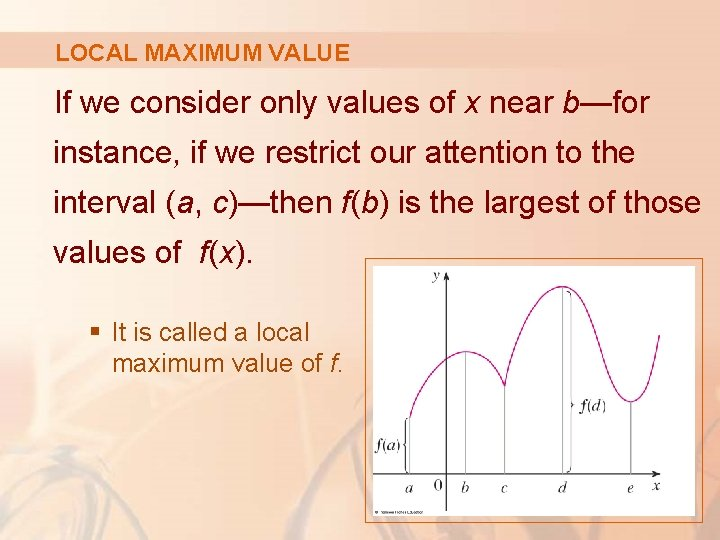LOCAL MAXIMUM VALUE If we consider only values of x near b—for instance, if we restrict our attention to the interval (a, c)—then f(b) is the largest of those values of f(x). § It is called a local maximum value of f.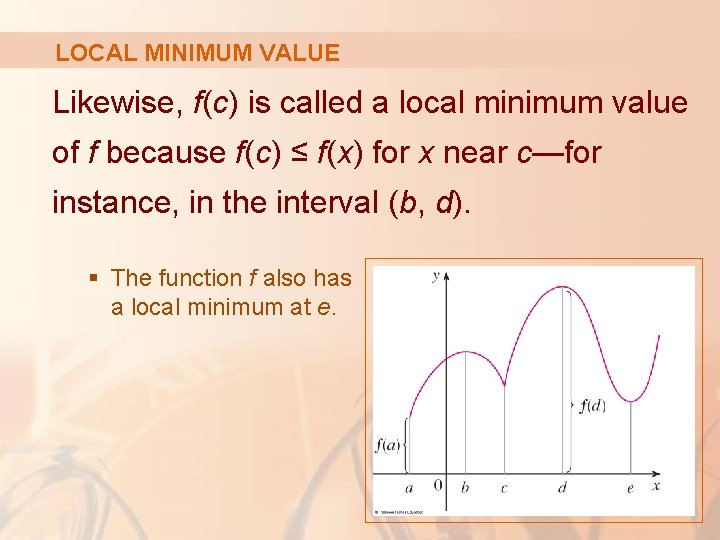LOCAL MINIMUM VALUE Likewise, f(c) is called a local minimum value of f because f(c) ≤ f(x) for x near c—for instance, in the interval (b, d). § The function f also has a local minimum at e.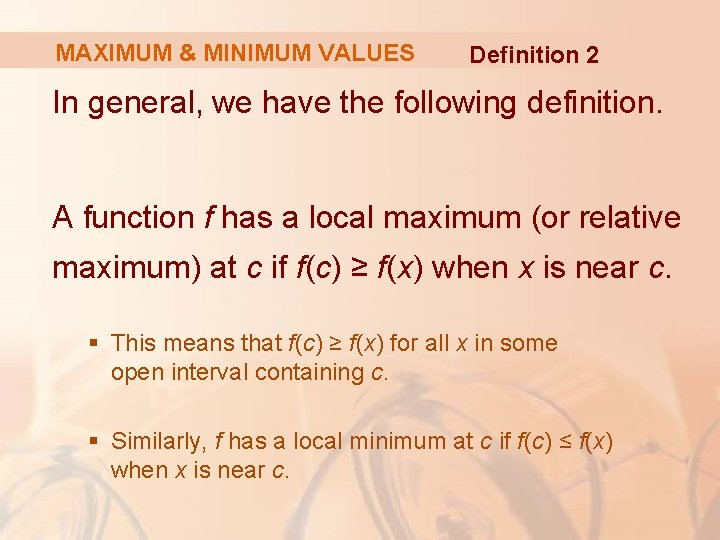MAXIMUM & MINIMUM VALUES Definition 2 In general, we have the following definition. A function f has a local maximum (or relative maximum) at c if f(c) ≥ f(x) when x is near c. § This means that f(c) ≥ f(x) for all x in some open interval containing c. § Similarly, f has a local minimum at c if f(c) ≤ f(x) when x is near c.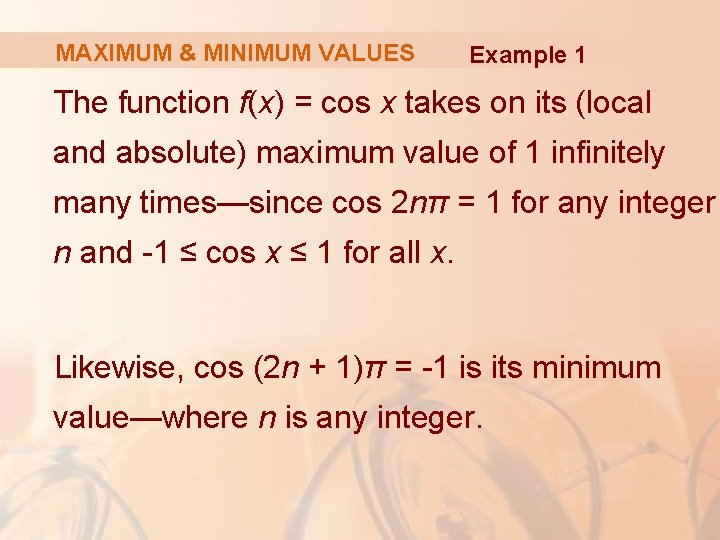MAXIMUM & MINIMUM VALUES Example 1 The function f(x) = cos x takes on its (local and absolute) maximum value of 1 infinitely many times—since cos 2 nπ = 1 for any integer n and -1 ≤ cos x ≤ 1 for all x. Likewise, cos (2 n + 1)π = -1 is its minimum value—where n is any integer.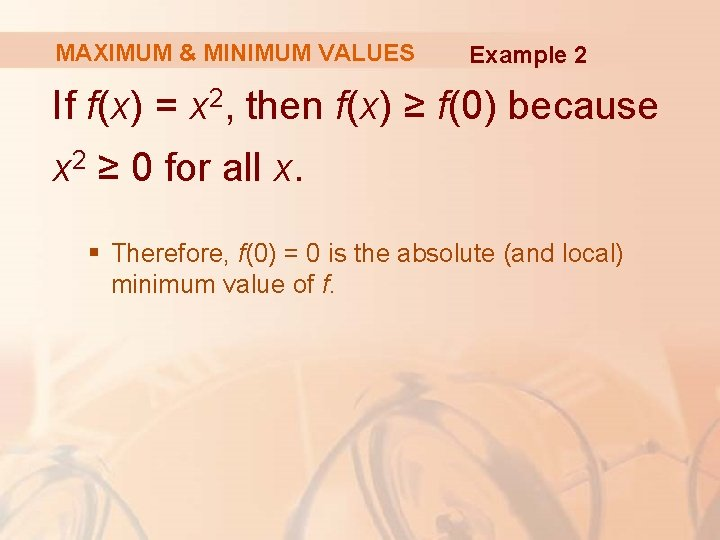MAXIMUM & MINIMUM VALUES Example 2 If f(x) = x 2, then f(x) ≥ f(0) because x 2 ≥ 0 for all x. § Therefore, f(0) = 0 is the absolute (and local) minimum value of f.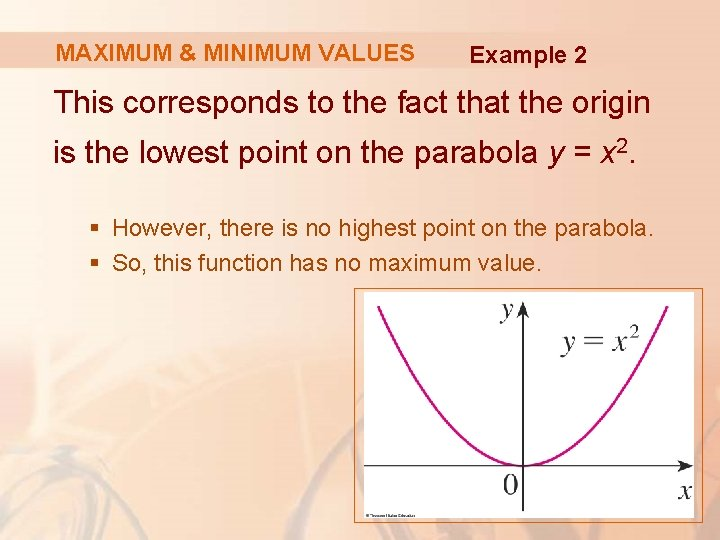MAXIMUM & MINIMUM VALUES Example 2 This corresponds to the fact that the origin is the lowest point on the parabola y = x 2. § However, there is no highest point on the parabola. § So, this function has no maximum value.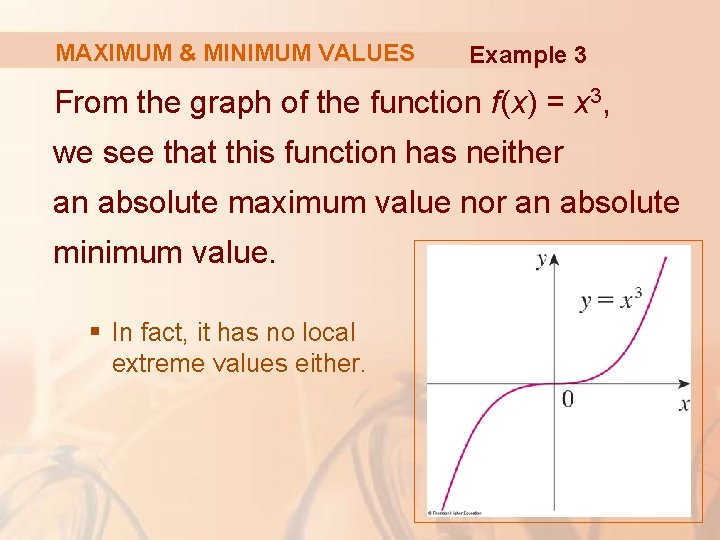MAXIMUM & MINIMUM VALUES Example 3 From the graph of the function f(x) = x 3, we see that this function has neither an absolute maximum value nor an absolute minimum value. § In fact, it has no local extreme values either.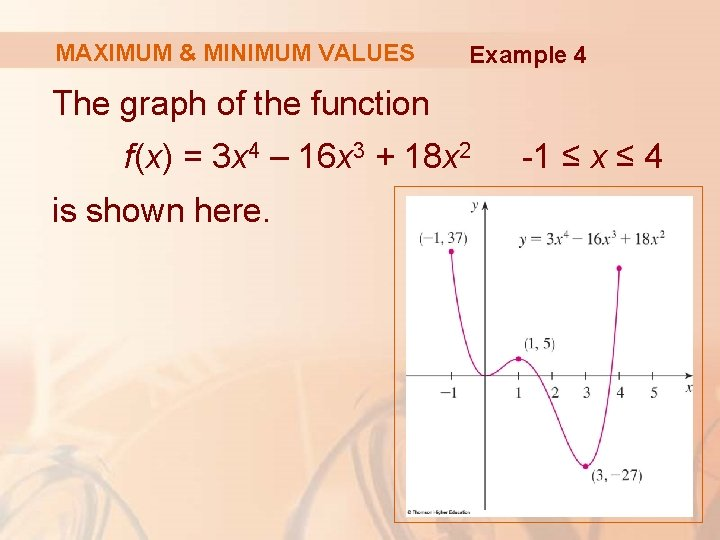MAXIMUM & MINIMUM VALUES Example 4 The graph of the function f(x) = 3 x 4 – 16 x 3 + 18 x 2 is shown here. -1 ≤ x ≤ 4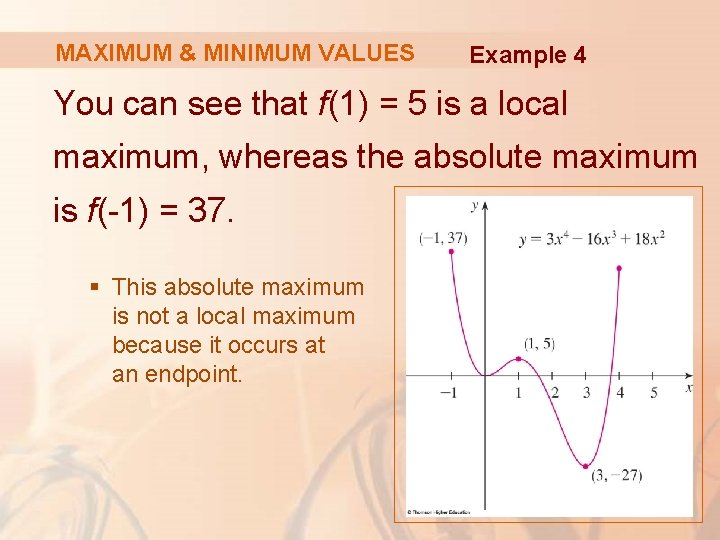MAXIMUM & MINIMUM VALUES Example 4 You can see that f(1) = 5 is a local maximum, whereas the absolute maximum is f(-1) = 37. § This absolute maximum is not a local maximum because it occurs at an endpoint.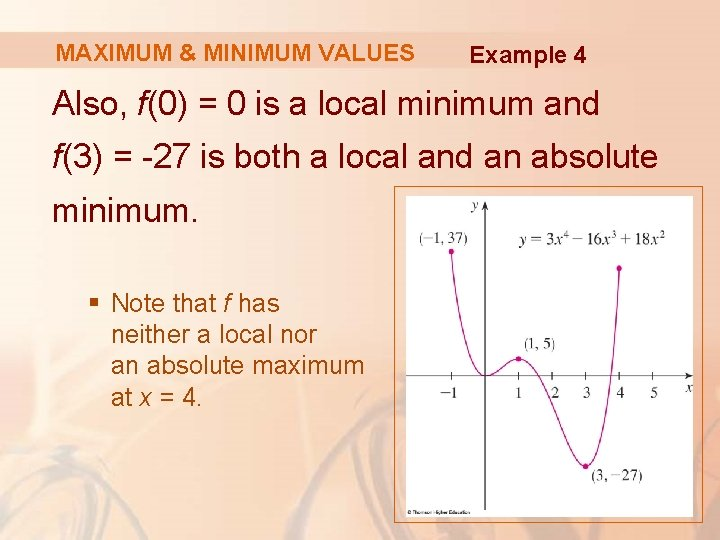MAXIMUM & MINIMUM VALUES Example 4 Also, f(0) = 0 is a local minimum and f(3) = -27 is both a local and an absolute minimum. § Note that f has neither a local nor an absolute maximum at x = 4.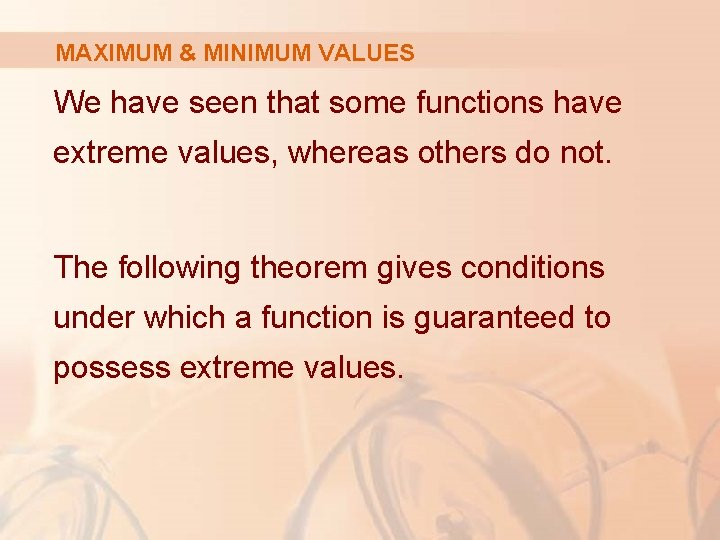MAXIMUM & MINIMUM VALUES We have seen that some functions have extreme values, whereas others do not. The following theorem gives conditions under which a function is guaranteed to possess extreme values.EXTREME VALUE THEOREM Theorem 3 If f is continuous on a closed interval [a, b], then f attains an absolute maximum value f(c) and an absolute minimum value f(d) at some numbers c and d in [a, b].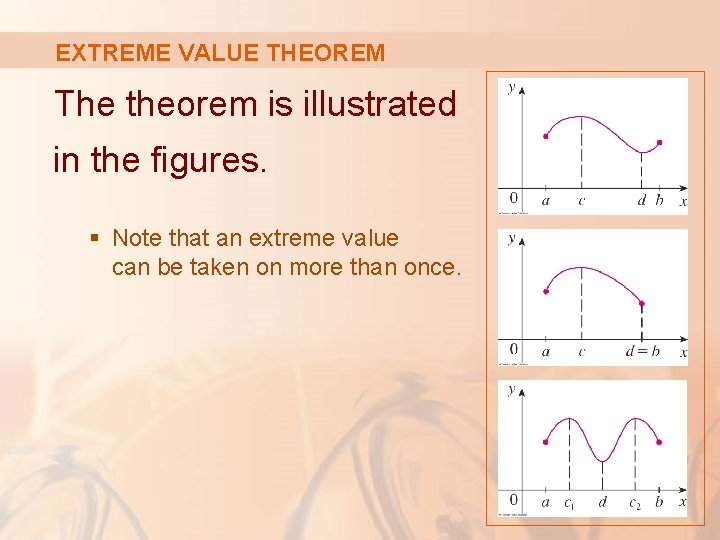EXTREME VALUE THEOREM The theorem is illustrated in the figures. § Note that an extreme value can be taken on more than once.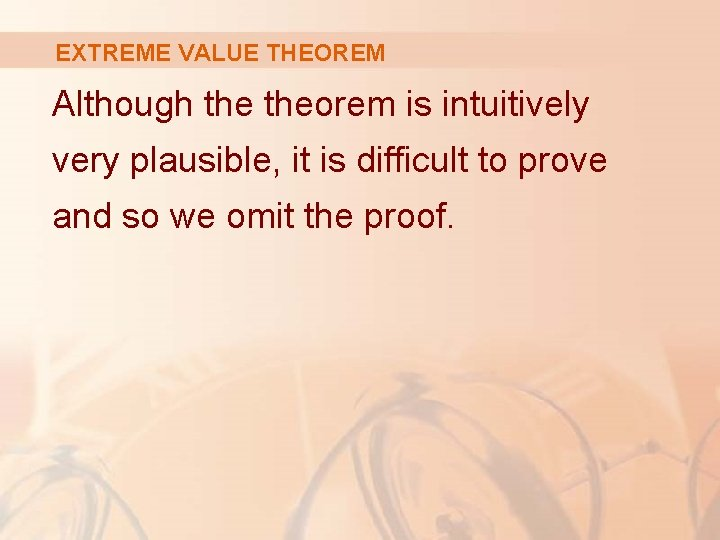EXTREME VALUE THEOREM Although theorem is intuitively very plausible, it is difficult to prove and so we omit the proof.EXTREME VALUE THEOREM The figures show that a function need not possess extreme values if either hypothesis (continuity or closed interval) is omitted from theorem.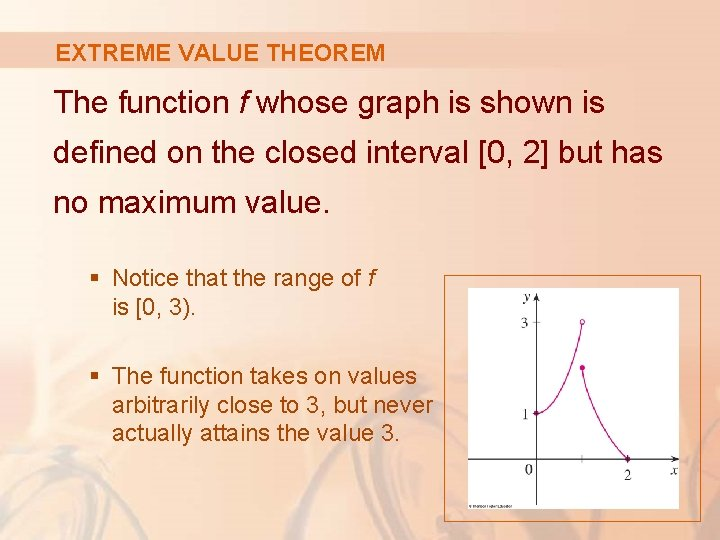EXTREME VALUE THEOREM The function f whose graph is shown is defined on the closed interval [0, 2] but has no maximum value. § Notice that the range of f is [0, 3). § The function takes on values arbitrarily close to 3, but never actually attains the value 3.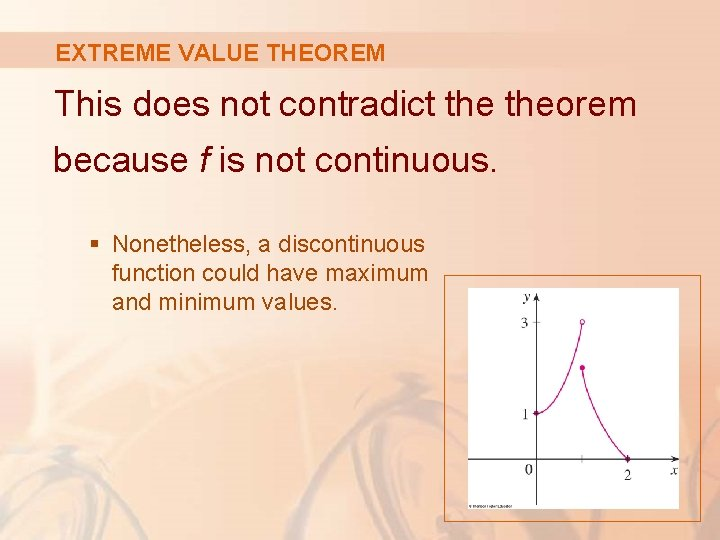EXTREME VALUE THEOREM This does not contradict theorem because f is not continuous. § Nonetheless, a discontinuous function could have maximum and minimum values.EXTREME VALUE THEOREM The function g shown here is continuous on the open interval (0, 2) but has neither a maximum nor a minimum value. § The range of g is (1, ∞). § The function takes on arbitrarily large values. § This does not contradict theorem because the interval (0, 2) is not closed.EXTREME VALUE THEOREM The theorem says that a continuous function on a closed interval has a maximum value and a minimum value. However, it does not tell us how to find these extreme values. § We start by looking for local extreme values.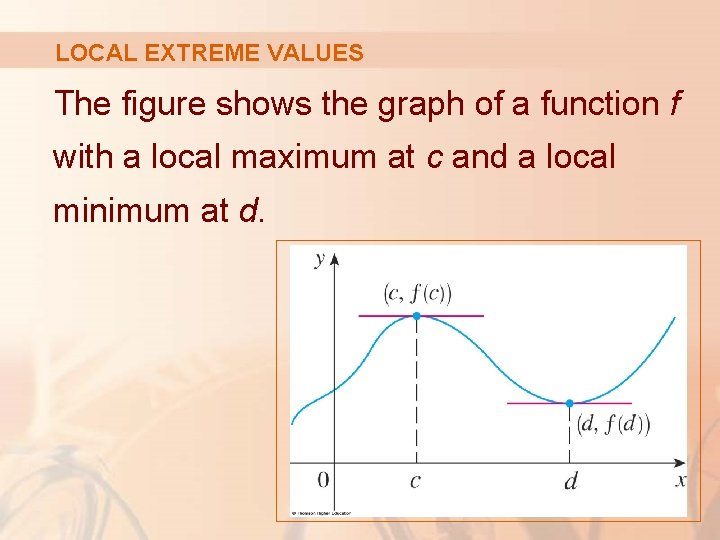LOCAL EXTREME VALUES The figure shows the graph of a function f with a local maximum at c and a local minimum at d.LOCAL EXTREME VALUES It appears that, at the maximum and minimum points, the tangent lines are horizontal and therefore each has slope 0.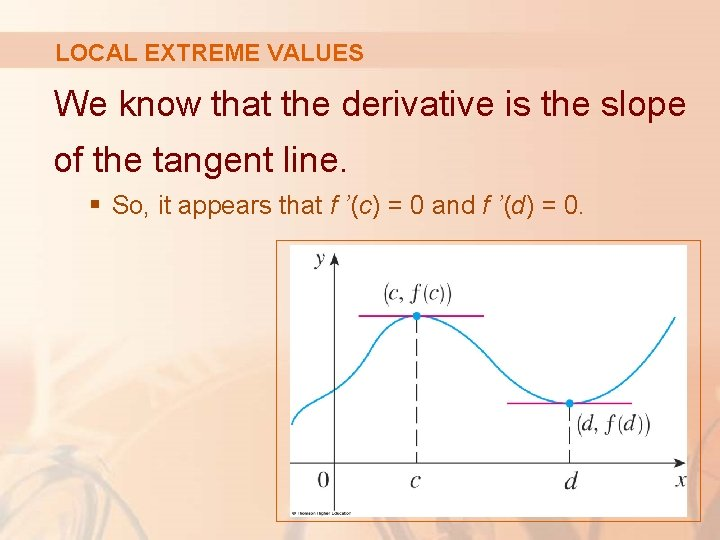LOCAL EXTREME VALUES We know that the derivative is the slope of the tangent line. § So, it appears that f ’(c) = 0 and f ’(d) = 0.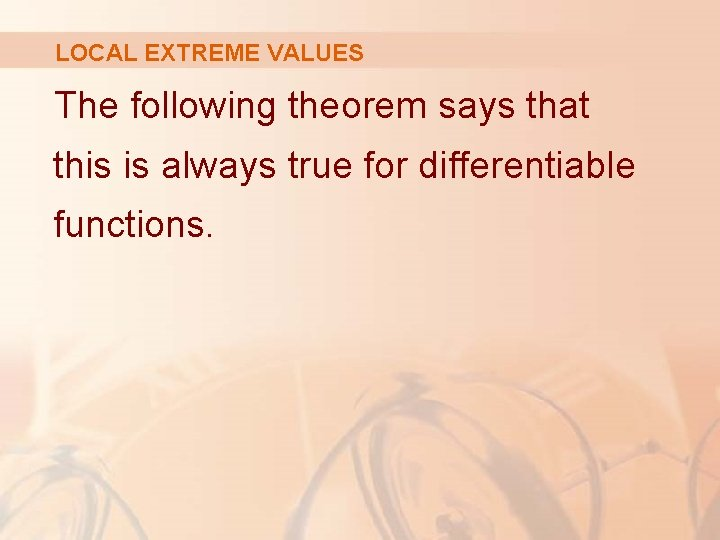LOCAL EXTREME VALUES The following theorem says that this is always true for differentiable functions.FERMAT’S THEOREM Theorem 4 If f has a local maximum or minimum at c, and if f ’(c) exists, then f ’(c) = 0.FERMAT’S THEOREM Proof Suppose, for the sake of definiteness, that f has a local maximum at c. Then, according to Definition 2, f(c) ≥ f(x) if x is sufficiently close to c.FERMAT’S THEOREM Proof (Equation 5) This implies that, if h is sufficiently close to 0, with h being positive or negative, then f(c) ≥ f(c + h) and therefore f(c + h) – f(c) ≤ 0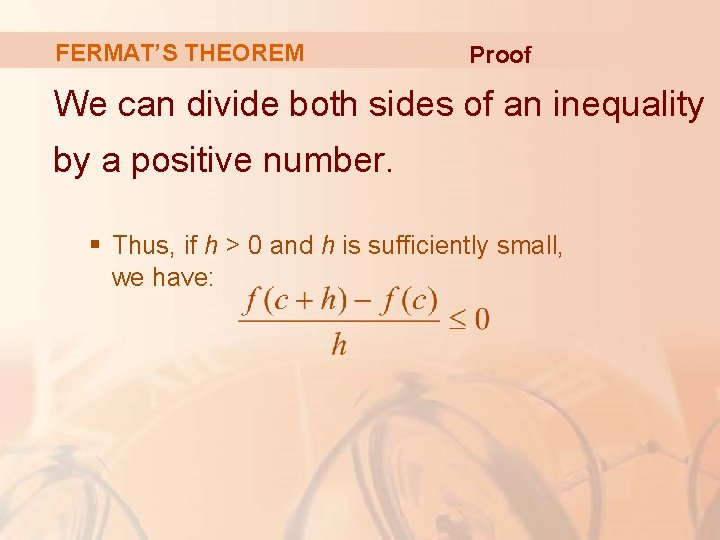FERMAT’S THEOREM Proof We can divide both sides of an inequality by a positive number. § Thus, if h > 0 and h is sufficiently small, we have: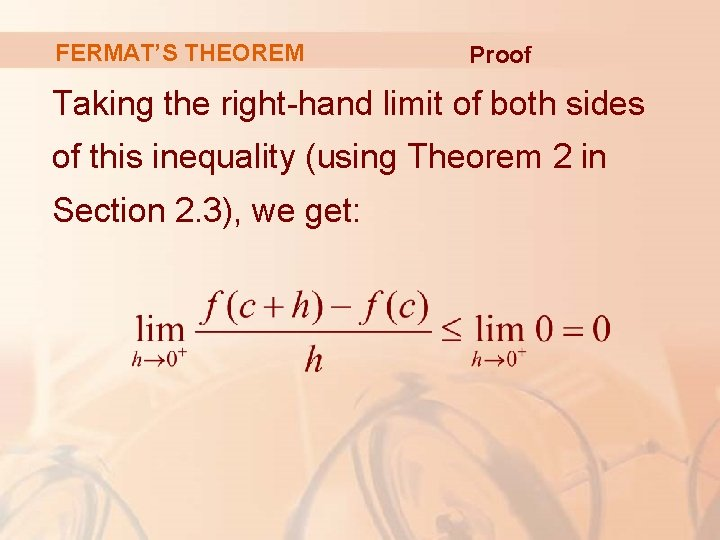FERMAT’S THEOREM Proof Taking the right-hand limit of both sides of this inequality (using Theorem 2 in Section 2. 3), we get: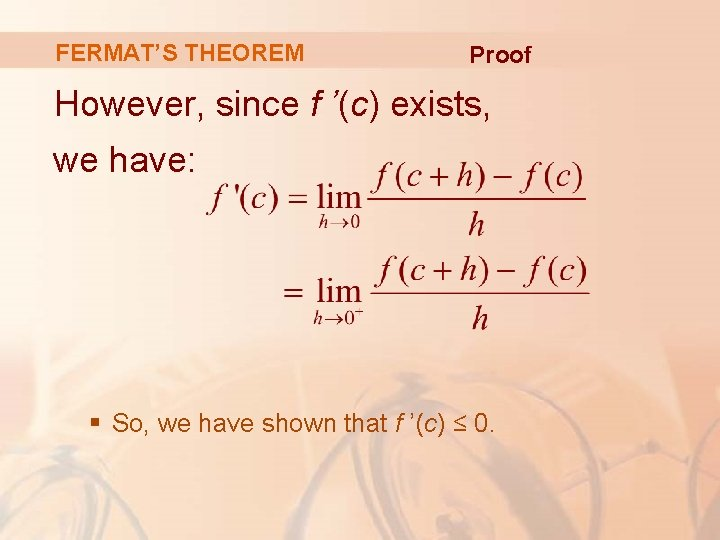FERMAT’S THEOREM Proof However, since f ’(c) exists, we have: § So, we have shown that f ’(c) ≤ 0.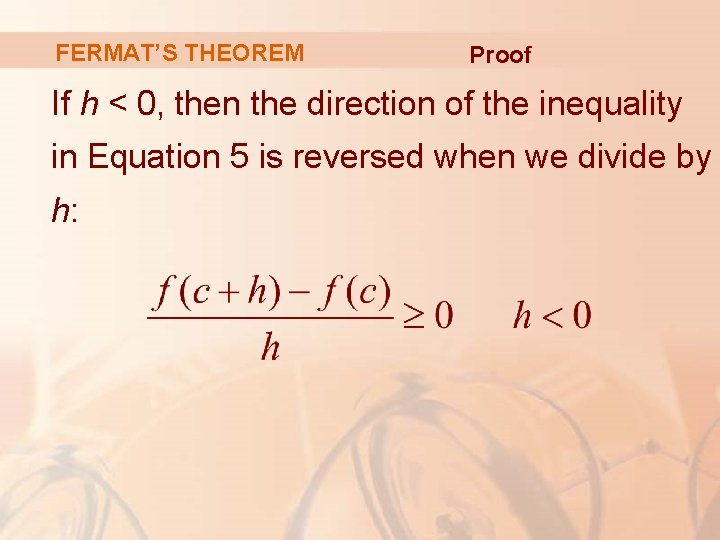FERMAT’S THEOREM Proof If h < 0, then the direction of the inequality in Equation 5 is reversed when we divide by h: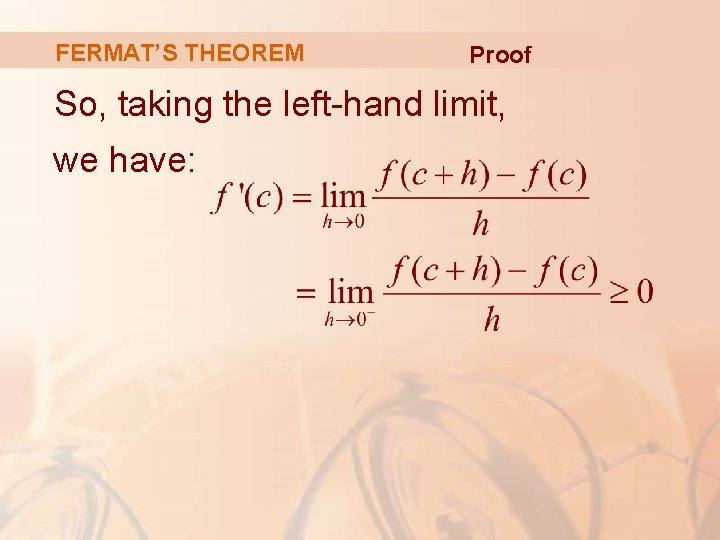FERMAT’S THEOREM Proof So, taking the left-hand limit, we have: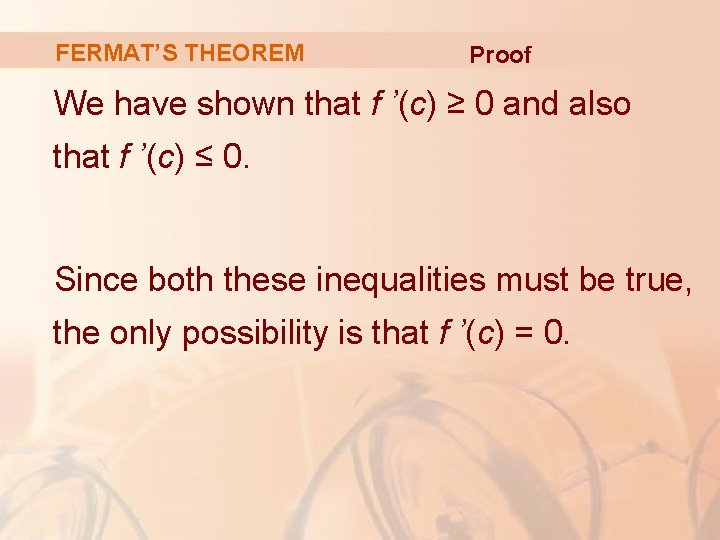FERMAT’S THEOREM Proof We have shown that f ’(c) ≥ 0 and also that f ’(c) ≤ 0. Since both these inequalities must be true, the only possibility is that f ’(c) = 0.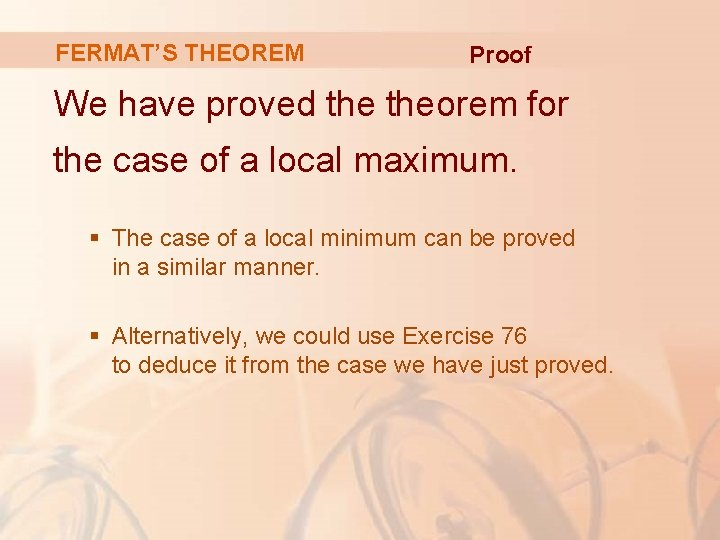FERMAT’S THEOREM Proof We have proved theorem for the case of a local maximum. § The case of a local minimum can be proved in a similar manner. § Alternatively, we could use Exercise 76 to deduce it from the case we have just proved.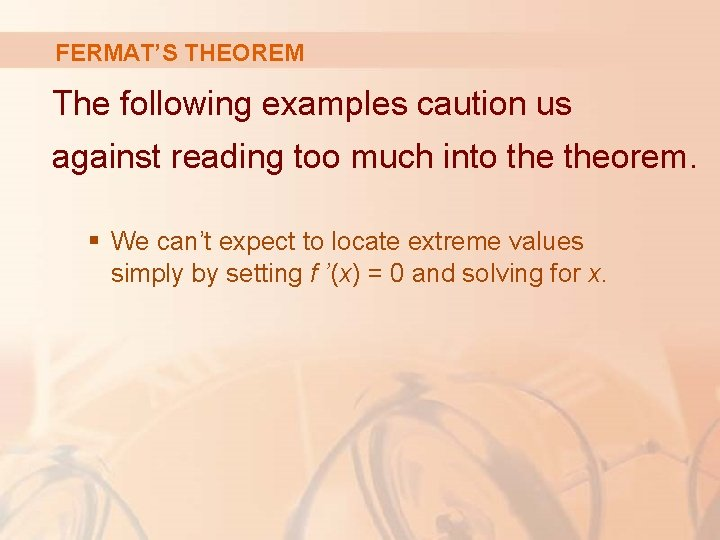FERMAT’S THEOREM The following examples caution us against reading too much into theorem. § We can’t expect to locate extreme values simply by setting f ’(x) = 0 and solving for x.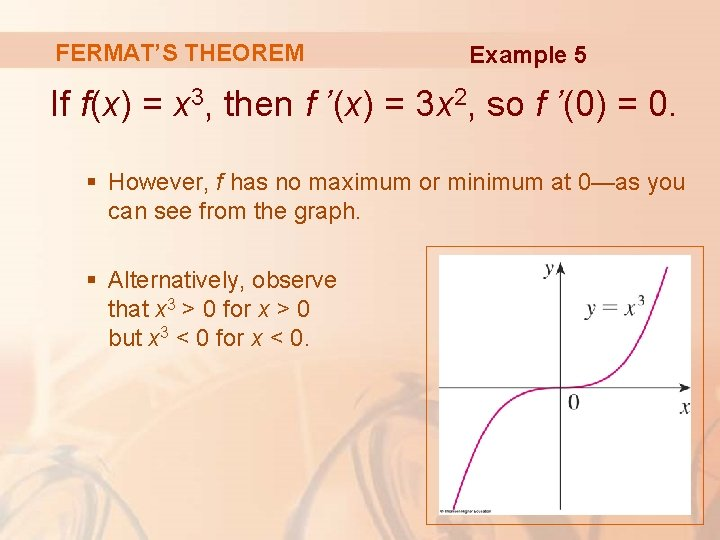FERMAT’S THEOREM Example 5 If f(x) = x 3, then f ’(x) = 3 x 2, so f ’(0) = 0. § However, f has no maximum or minimum at 0—as you can see from the graph. § Alternatively, observe that x 3 > 0 for x > 0 but x 3 < 0 for x < 0.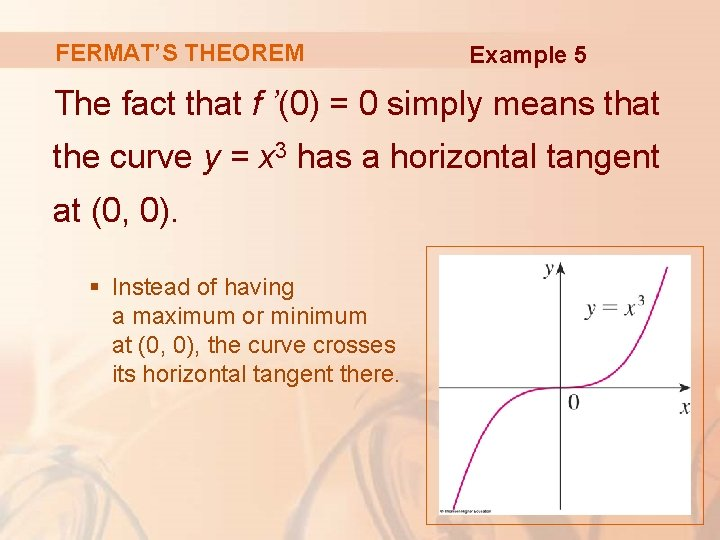FERMAT’S THEOREM Example 5 The fact that f ’(0) = 0 simply means that the curve y = x 3 has a horizontal tangent at (0, 0). § Instead of having a maximum or minimum at (0, 0), the curve crosses its horizontal tangent there.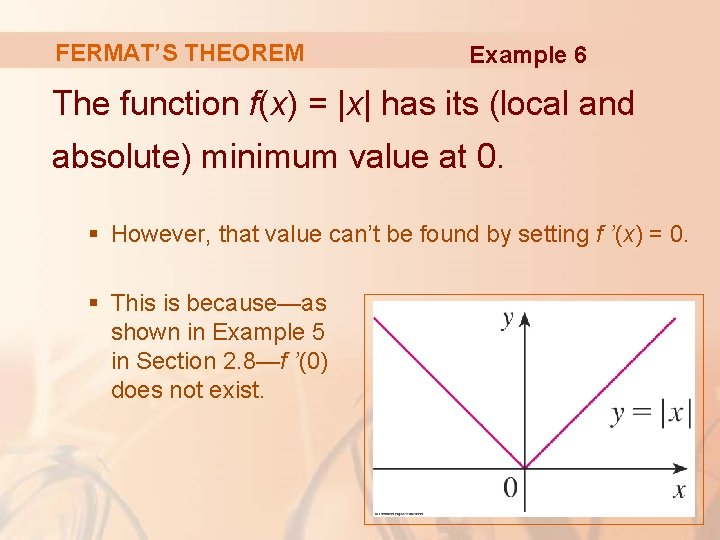FERMAT’S THEOREM Example 6 The function f(x) = |x| has its (local and absolute) minimum value at 0. § However, that value can’t be found by setting f ’(x) = 0. § This is because—as shown in Example 5 in Section 2. 8—f ’(0) does not exist.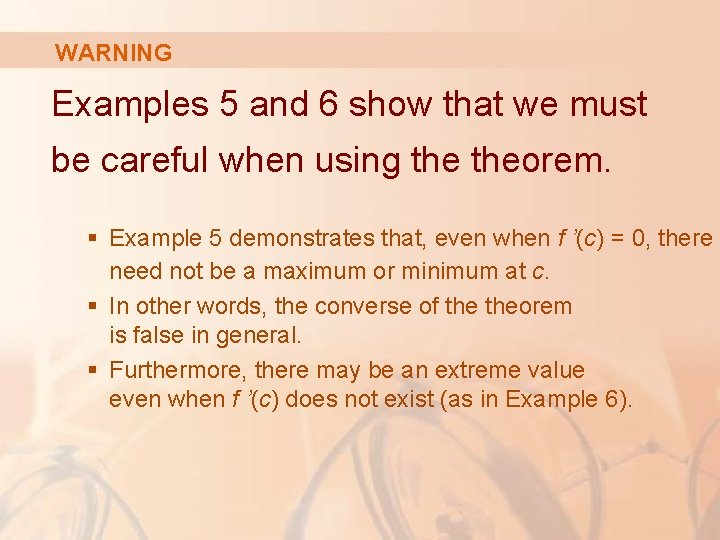WARNING Examples 5 and 6 show that we must be careful when using theorem. § Example 5 demonstrates that, even when f ’(c) = 0, there need not be a maximum or minimum at c. § In other words, the converse of theorem is false in general. § Furthermore, there may be an extreme value even when f ’(c) does not exist (as in Example 6).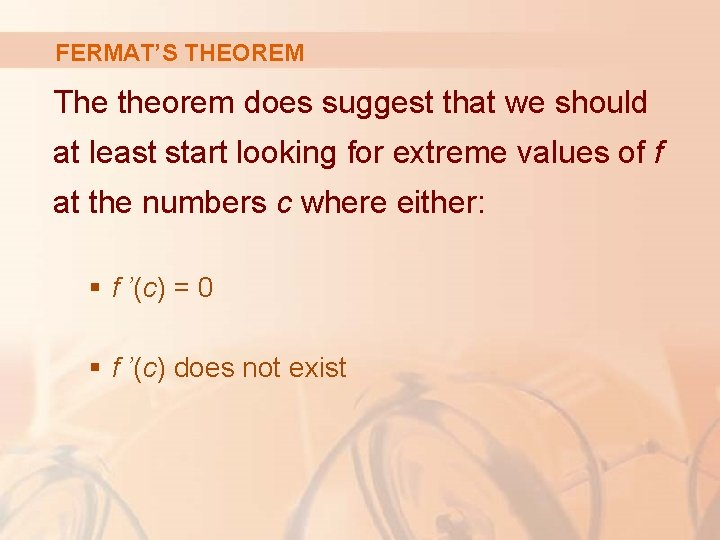FERMAT’S THEOREM The theorem does suggest that we should at least start looking for extreme values of f at the numbers c where either: § f ’(c) = 0 § f ’(c) does not existFERMAT’S THEOREM Such numbers are given a special name—critical numbers.CRITICAL NUMBERS Definition 6 A critical number of a function f is a number c in the domain of f such that either f ’(c) = 0 or f ’(c) does not exist.CRITICAL NUMBERS Example 7 Find the critical numbers of f(x) = x 3/5(4 - x). § The Product Rule gives: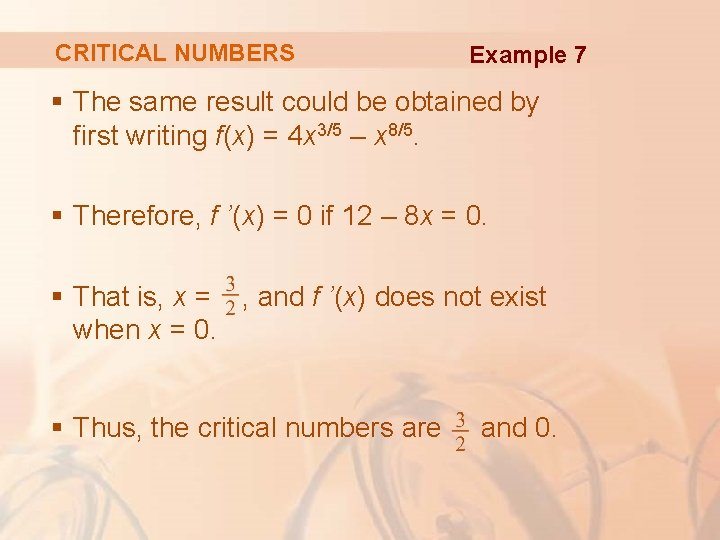CRITICAL NUMBERS Example 7 § The same result could be obtained by first writing f(x) = 4 x 3/5 – x 8/5. § Therefore, f ’(x) = 0 if 12 – 8 x = 0. § That is, x = , and f ’(x) does not exist when x = 0. § Thus, the critical numbers are and 0.CRITICAL NUMBERS In terms of critical numbers, Fermat’s Theorem can be rephrased as follows (compare Definition 6 with Theorem 4).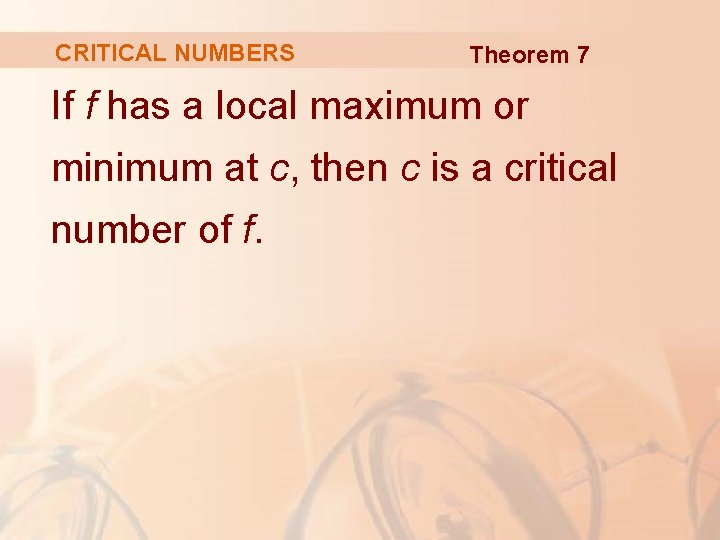CRITICAL NUMBERS Theorem 7 If f has a local maximum or minimum at c, then c is a critical number of f.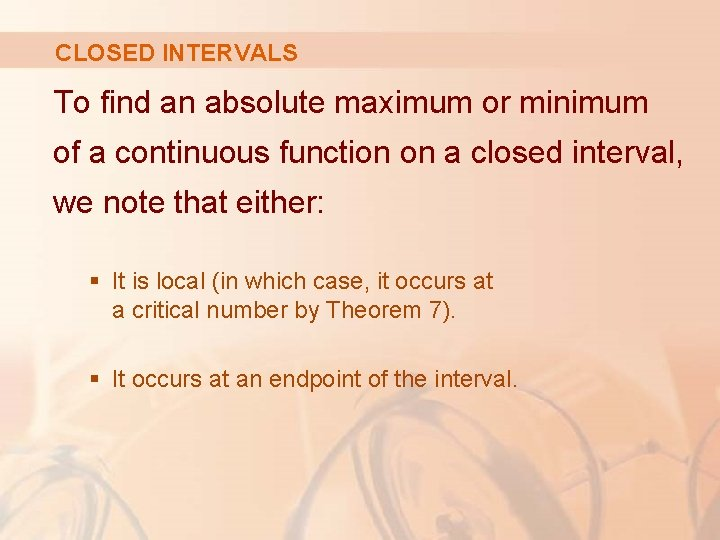CLOSED INTERVALS To find an absolute maximum or minimum of a continuous function on a closed interval, we note that either: § It is local (in which case, it occurs at a critical number by Theorem 7). § It occurs at an endpoint of the interval.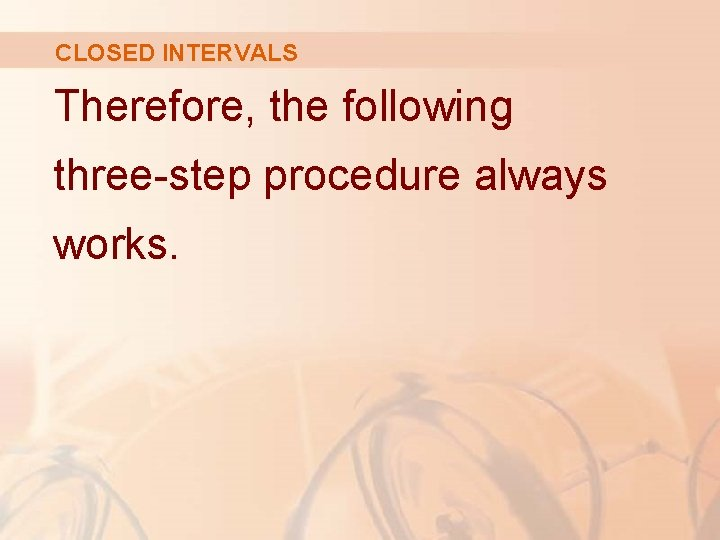CLOSED INTERVALS Therefore, the following three-step procedure always works.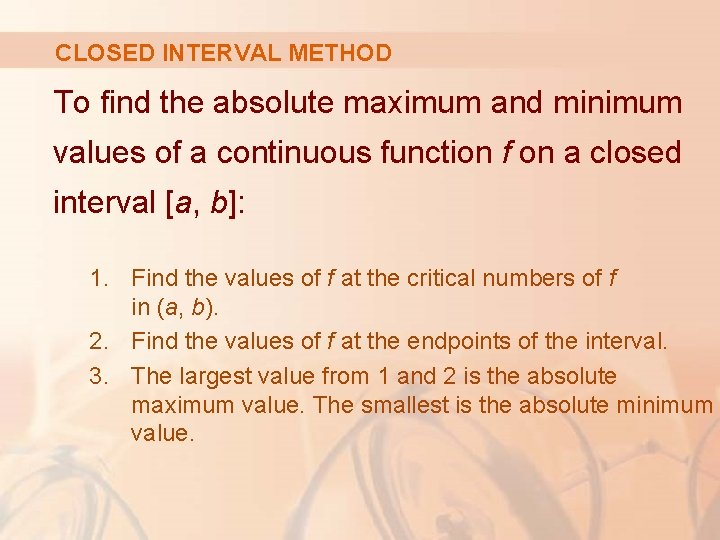CLOSED INTERVAL METHOD To find the absolute maximum and minimum values of a continuous function f on a closed interval [a, b]: 1. Find the values of f at the critical numbers of f in (a, b). 2. Find the values of f at the endpoints of the interval. 3. The largest value from 1 and 2 is the absolute maximum value. The smallest is the absolute minimum value.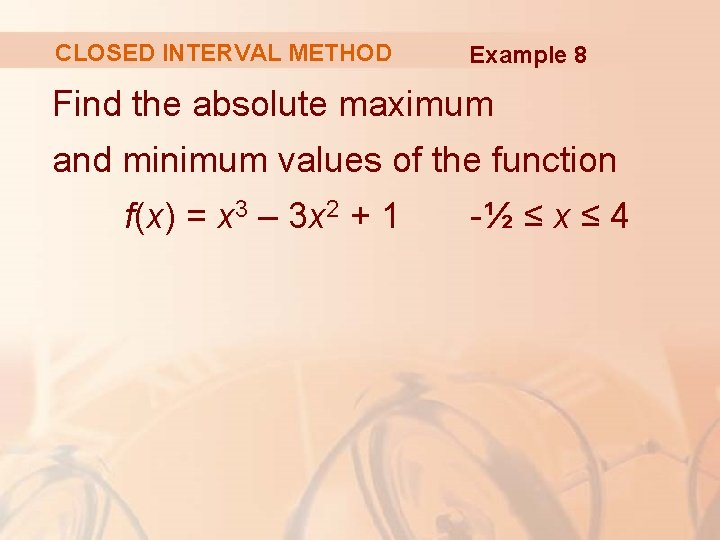CLOSED INTERVAL METHOD Example 8 Find the absolute maximum and minimum values of the function f(x) = x 3 – 3 x 2 + 1 -½ ≤ x ≤ 4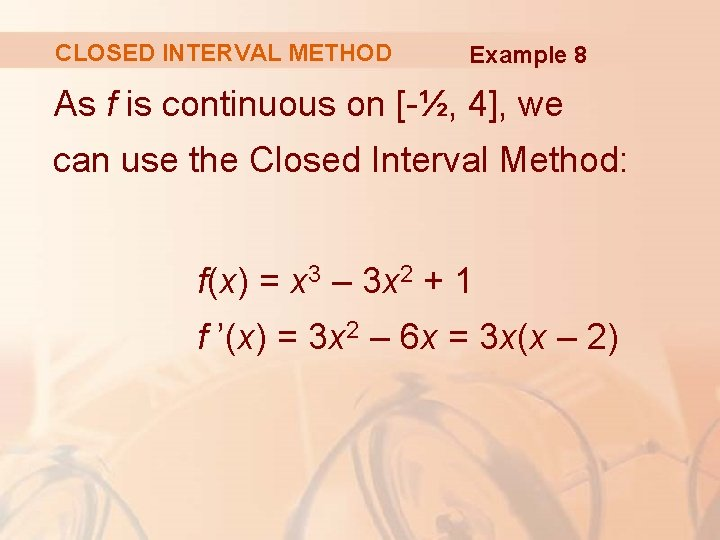CLOSED INTERVAL METHOD Example 8 As f is continuous on [-½, 4], we can use the Closed Interval Method: f(x) = x 3 – 3 x 2 + 1 f ’(x) = 3 x 2 – 6 x = 3 x(x – 2)CLOSED INTERVAL METHOD Example 8 As f ’(x) exists for all x, the only critical numbers of f occur when f ’(x) = 0, that is, x = 0 or x = 2. Notice that each of these numbers lies in the interval (-½, 4).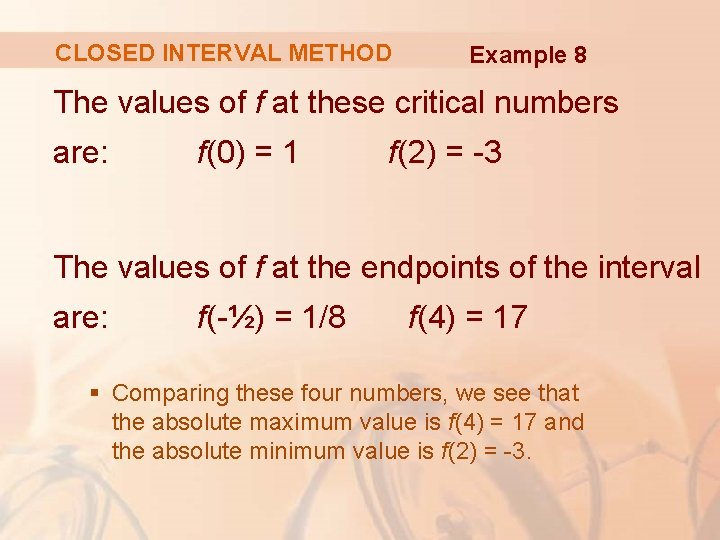CLOSED INTERVAL METHOD Example 8 The values of f at these critical numbers are: f(0) = 1 f(2) = -3 The values of f at the endpoints of the interval are: f(-½) = 1/8 f(4) = 17 § Comparing these four numbers, we see that the absolute maximum value is f(4) = 17 and the absolute minimum value is f(2) = -3.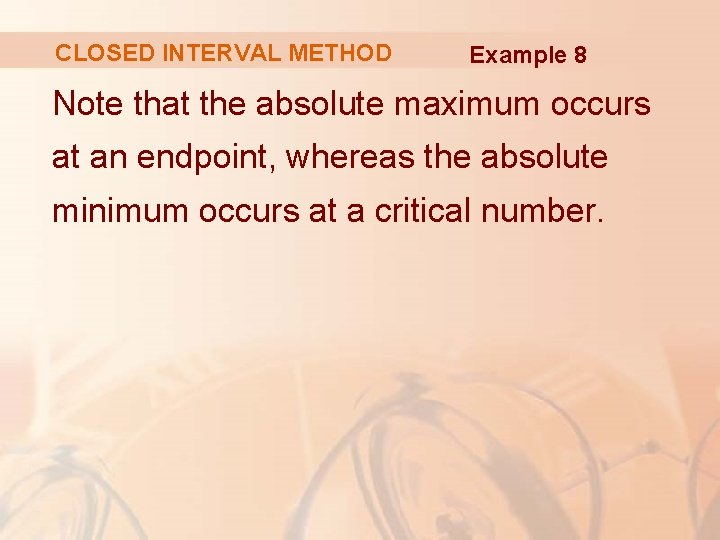CLOSED INTERVAL METHOD Example 8 Note that the absolute maximum occurs at an endpoint, whereas the absolute minimum occurs at a critical number.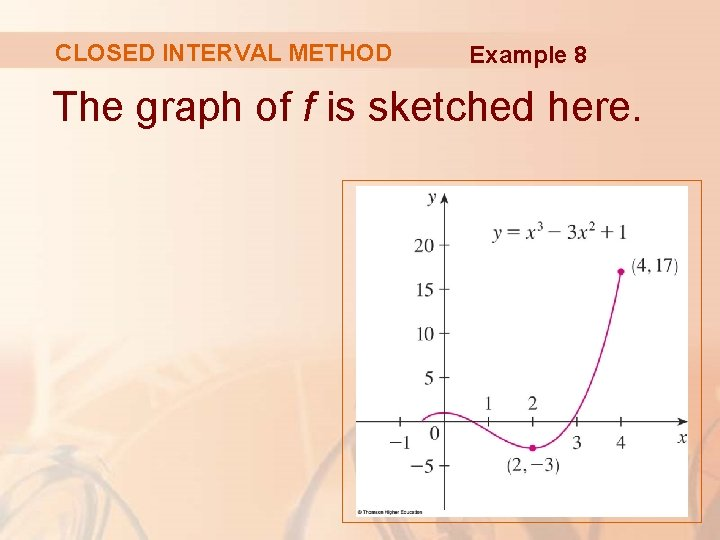CLOSED INTERVAL METHOD Example 8 The graph of f is sketched here.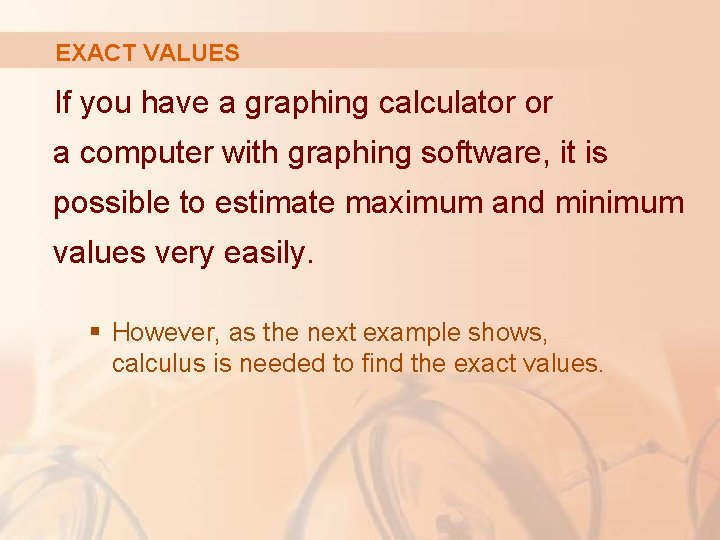EXACT VALUES If you have a graphing calculator or a computer with graphing software, it is possible to estimate maximum and minimum values very easily. § However, as the next example shows, calculus is needed to find the exact values.EXACT VALUES Example 9 a. Use a graphing device to estimate the absolute minimum and maximum values of the function f(x) = x – 2 sin x, 0 ≤ x ≤ 2π. b. Use calculus to find the exact minimum and maximum values.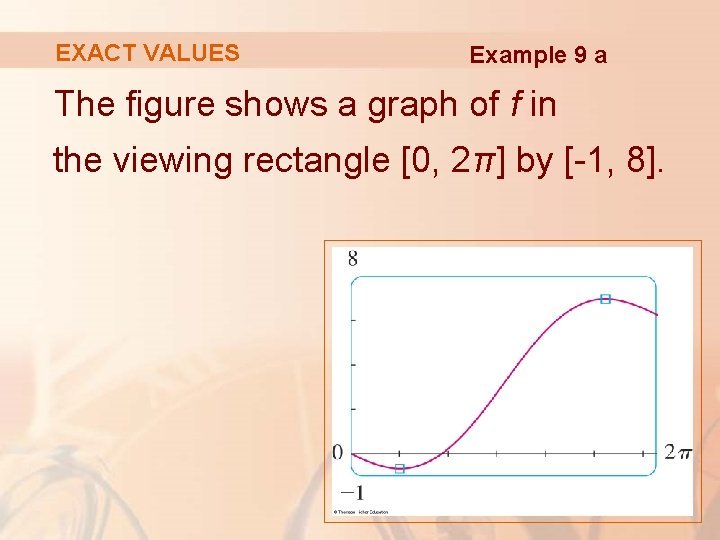EXACT VALUES Example 9 a The figure shows a graph of f in the viewing rectangle [0, 2π] by [-1, 8].EXACT VALUES Example 9 a By moving the cursor close to the maximum point, we see the y-coordinates don’t change very much in the vicinity of the maximum. § The absolute maximum value is about 6. 97 § It occurs when x ≈ 5. 2EXACT VALUES Example 9 a Similarly, by moving the cursor close to the minimum point, we see the absolute minimum value is about – 0. 68 and it occurs when x ≈ 1. 0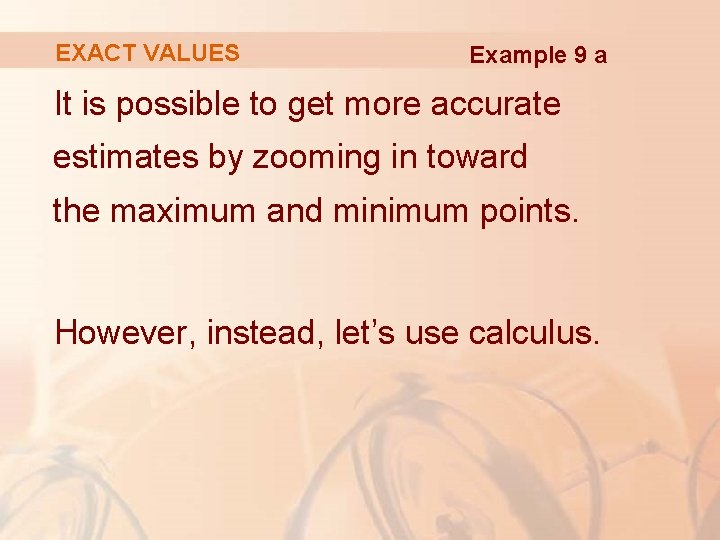EXACT VALUES Example 9 a It is possible to get more accurate estimates by zooming in toward the maximum and minimum points. However, instead, let’s use calculus.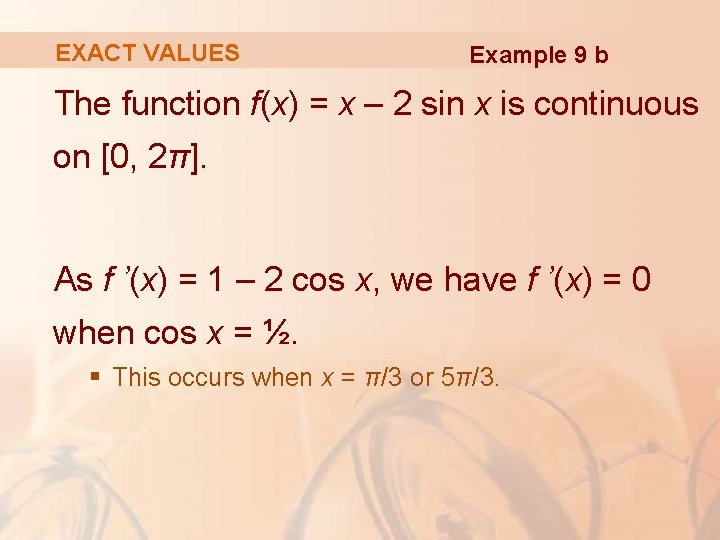EXACT VALUES Example 9 b The function f(x) = x – 2 sin x is continuous on [0, 2π]. As f ’(x) = 1 – 2 cos x, we have f ’(x) = 0 when cos x = ½. § This occurs when x = π/3 or 5π/3.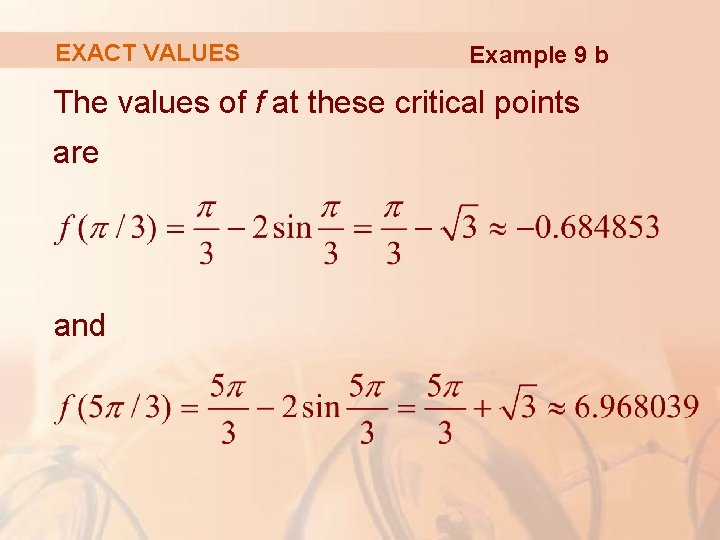EXACT VALUES Example 9 b The values of f at these critical points are and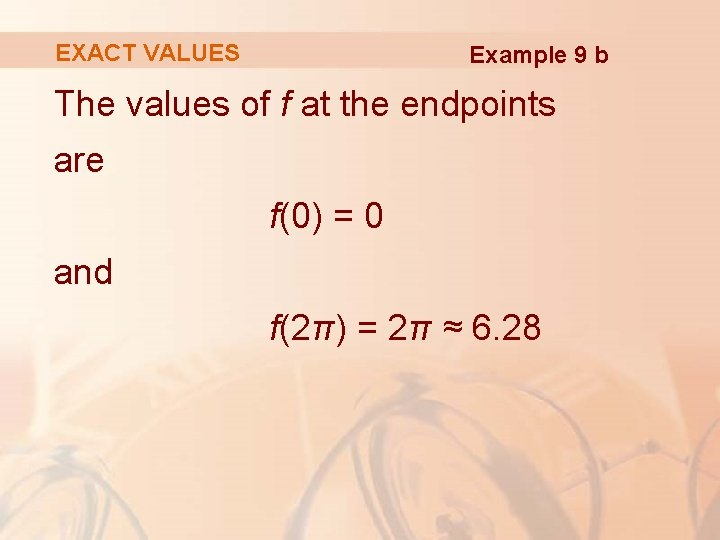EXACT VALUES Example 9 b The values of f at the endpoints are f(0) = 0 and f(2π) = 2π ≈ 6. 28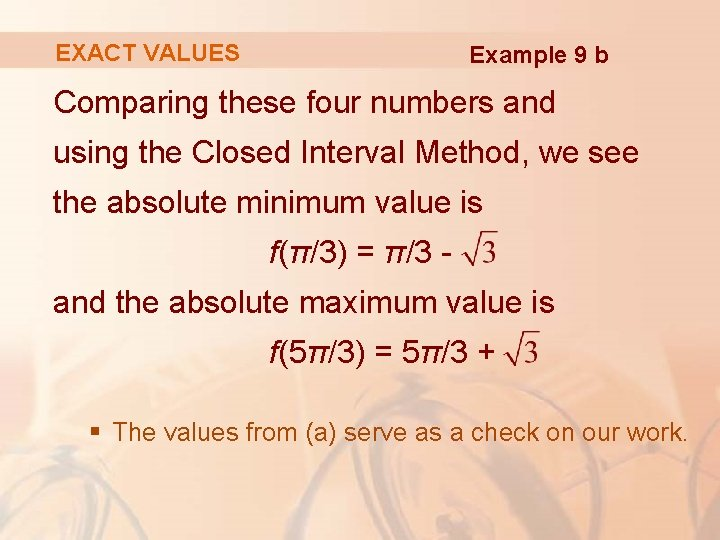EXACT VALUES Example 9 b Comparing these four numbers and using the Closed Interval Method, we see the absolute minimum value is f(π/3) = π/3 and the absolute maximum value is f(5π/3) = 5π/3 + § The values from (a) serve as a check on our work.MAXIMUM & MINIMUM VALUES Example 10 The Hubble Space Telescope was deployed on April 24, 1990, by the space shuttle Discovery.MAXIMUM & MINIMUM VALUES Example 10 A model for the velocity of the shuttle during this mission—from liftoff at t = 0 until the solid rocket boosters were jettisoned at t = 126 s—is given by: v(t) = 0. 001302 t 3 – 0. 09029 t 2 + 23. 61 t – 3. 083 (in feet per second)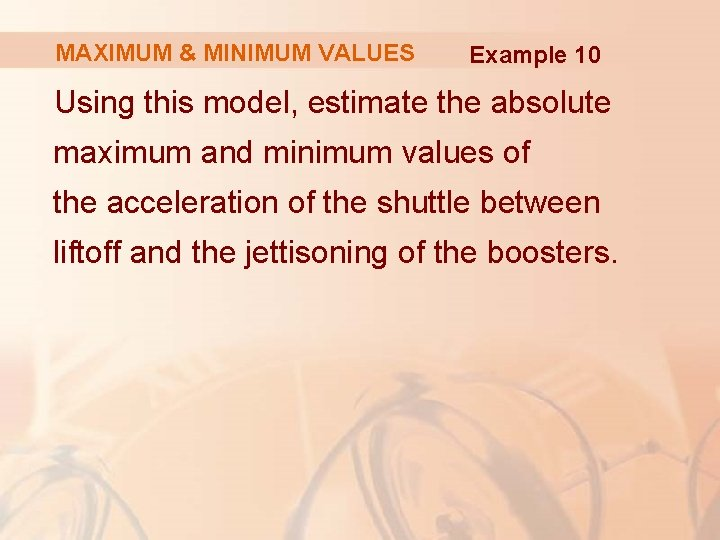MAXIMUM & MINIMUM VALUES Example 10 Using this model, estimate the absolute maximum and minimum values of the acceleration of the shuttle between liftoff and the jettisoning of the boosters.MAXIMUM & MINIMUM VALUES Example 10 We are asked for the extreme values not of the given velocity function, but rather of the acceleration function.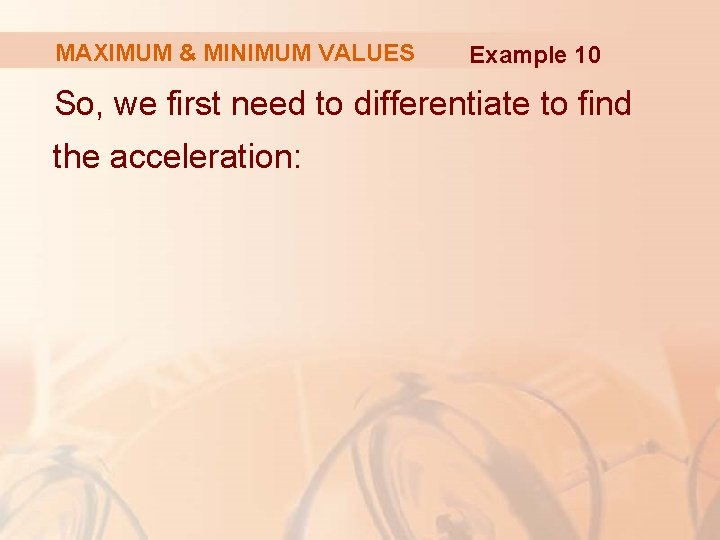MAXIMUM & MINIMUM VALUES Example 10 So, we first need to differentiate to find the acceleration:MAXIMUM & MINIMUM VALUES Example 10 We now apply the Closed Interval Method to the continuous function a on the interval 0 ≤ t ≤ 126. Its derivative is: a’(t) = 0. 007812 t – 0. 18058MAXIMUM & MINIMUM VALUES Example 10 The only critical number occurs when a’(t) = 0: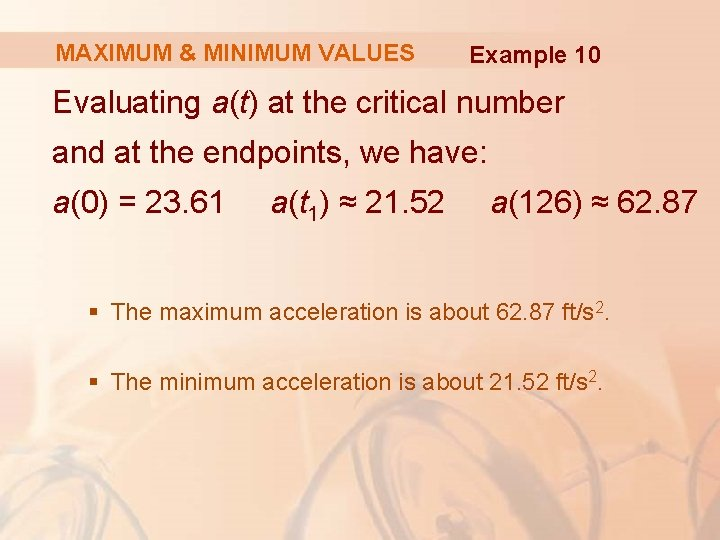MAXIMUM & MINIMUM VALUES Example 10 Evaluating a(t) at the critical number and at the endpoints, we have: a(0) = 23. 61 a(t 1) ≈ 21. 52 a(126) ≈ 62. 87 § The maximum acceleration is about 62. 87 ft/s 2. § The minimum acceleration is about 21. 52 ft/s 2.